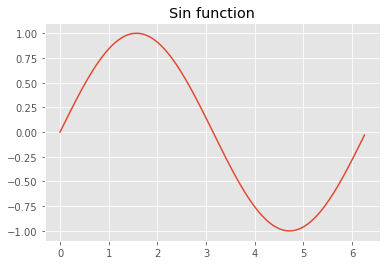# Simple Sin Function

This is a simple illustration that the
• code highlighting works
• plots are shown
This post that was exported from a Jupyiter notbook, as described in the previous post Introduction and Hello!
We start with importing numpy and matplotlib, two important python packages.

Now we generate vectorised x and y values for plotting:So,  it works, hurray!